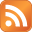# Command and Conquer: Red Alert Retaliation Playstation cheatsCheat codes

Atomic Bomb
Access the sidebar menu, then highlight the symbols below in the order as shown and press circle at each one.
CIRCLE, X, CIRCLE, CIRCLE, X, SQUARE

Chronoshift
Access the sidebar menu, then highlight the symbols below in the order as shown and press circle at each one.
SQUARE, CIRCLE, TRIANGLE, X, CIRCLE, CIRCLE

Equip Iron Curtain
In the middle of the game, press Triangle button to bring up the sidebar. Now, move the cursor over the appropriate symbols (X, Circle, Triangle, Square) and key in each sequence with the circle button to obtain the result.
Square, X, Circle, X, Triangle, Triangle

Equip Parabomb
In the middle of the game, press Triangle button to bring up the sidebar. Now, move the cursor over the appropriate symbols (X, Circle, Triangle, Square) and key in each sequence with the circle button to obtain the result.
X, X, X, Circle, Triangle, Square

Give Civilians Names
During a game, press triangle to enter the in-game sidebar, and then highlight the following sequence while pushing circle after each highlighted symbol:
Square, square, circle, circle, triangle, triangle.

Harvest People, Not Ore
During a game, press triangle to enter the in-game sidebar, and then highlight the following sequence while pushing circle after each highlighted symbol:
Square, X, square, X, square, X.

Increase Money
Press the triangle to bring up the sidebar. Move the cursor over the symbols and key in each sequence with the Circle button:
X, X, Square, Circle, Circle, Circle.
This will increase your cash by \$1,000 each time.

Lose the Current Mission
During a game, press triangle to enter the in-game sidebar, and then highlight the following sequence while pushing circle after each highlighted symbol:
Circle, X, circle, square, square, X.

More Codes
Full Map
1. Highlight triangle & press O
2. Highlight triangle
3. Highlight X & press O
4. Highlight O & press O
5. Highlight triangle & press O
6. Highlight square & press O

Nuclear Attack
1. Highlight O & press O
2. Highlight X & press O
3. Highlight O
4. Highlight O and press O
5. Highlight X and press O
6. Highlight square and press O

Parabomb
1. Highlight X and press O
2. Highlight X and press O
3. Highlight X and press O
4. Highlight O and press O
5. Highlight triangle and press O
6. Highlight square and press O

Reveal Entire Map
Access the sidebar menu, then highlight the symbols below in the order as shown and press circle at each one.
TRIANGLE, TRIANGLE, X, CIRCLE, TRIANGLE, SQUARE

Win Current Mission
In the middle of the game, press the Triangle to bring up the side bar, move the cursor over the following symbols and key in the sequence with the Circle button:
Circle, Circle, Triangle, X, X, Square